Disclaimer: This is an example of a student written essay.

Any scientific information contained within this essay should not be treated as fact, this content is to be used for educational purposes only and may contain factual inaccuracies or be out of date.

# Genetic Algorithm Research Proposal

 ✅ Paper Type: Free Essay ✅ Subject: Biology ✅ Wordcount: 1902 words ✅ Published: 31st Jul 2018

## System Optimization

The main concern is to find the optimum value for each design parameter for each prediction period for a total simulation time of 12 hours. The simulation is performed on the selected system based on the optimization timeframe with an acceptable accuracy and the optimization process is applied for a prediction period of one. The value of a single design parameter and internal loads are fixed during a prediction period and may vary from one prediction period to another.

If you need assistance with writing your essay, our professional essay writing service is here to help!

## Genetic Algorithm

Modeling the liquid desiccant system with the CC/DV system is complex task with multi-variables involved, several equations are coupled and indirect relations between different parameters are present. Since several non-linear equations are solved, it is advised to use a revolutionary derivative free optimization tool that follows the direct search technique. The simplest optimization tool that could be used for the proposed case is the genetic algorithm optimization tool because it is derivative free, based on numerical analysis, and is somehow efficient if compared with other derivative based optimization schemes. Moreover, it fetches the global minimum of a specific function.

Our choice of using a derivative free algorithm to solve the optimization problem is implemented by the evolutionary genetic algorithm. Genetic algorithms are adaptive methods which may be used to solve search and optimization problems, and are based on the genetic process of biological organisms. Genetic algorithms are growing more and more popular and extending from simple design optimization to online process control. The power of the genetic algorithm arises from its robustness, being acceptably good in finding the near optimum solution and being relatively quick . An efficient optimization technique uses two techniques to find the optimal solution, exploration and exploitation, and this is what genetic algorithm does.

### The Genetic Algorithm terminology

• The algorithm starts by seeding a set of trial combinations of the variables to be optimized and calculating the numerical value of the objective function for each combination selected. This set is called the “Initial Population”.
• The set of numerical values calculated for the objective function from the first trial, is then evaluated according the “Fitness Criteria. The fitness criteria can be defined as the condition for the objective function numerical value to be better convenient than its pears.
• Based on their fitness, some combinations in the previously seeded set are chosen to be “Parents”. Parents then undergo either “Crossover or “Mutation procedure to produce “Children”. Most fitted parents simply jump to the next generated population without any change; such parents are referred as “Elite.
• The current population is replaced by children from the next population.
• Elite children are the individuals in the current generation with the best fitness values. These individuals automatically survive to the next generation. Crossover children are created by combining the vectors of a pair of parents. Mutation children are created by introducing random changes, or mutations, to a single parent.
• The algorithm stops when the “Tolerance” in the objective function values between two generations is less than a certain set error value, or when the maximum number of “Generations” is exceeded, or by any other defined Stopping Criteria.

For the optimized control strategy used for the chilled ceiling, displacement ventilation system the variables of the chilled ceiling and displacement ventilation are varied; this variation leads to a minimal optimal cost that results in the minimum cost that could be attained in the system.

Referring to the system figure and considering the optimal control strategy, the variables that may be used for cost optimization are:

1. The desiccant temperature at the inlet of membrane().
2. The supply air temperature().
3. The supply air mass flow rate().

Equation Chapter 6 Section 3Each variable in the optimization routine has a lower and an upper bound. These bounds define the interval where the genetic algorithm searches for the optimal cost and are based on physical considerations. The bounds for the different variables according to ASHRAE’s recommendations are:

• The supply air temperature is considered to vary between 17 and 23 °C.
• The supply air mass flow rate is considered to vary between 0.08 and 0.26 kg/s.

## Optimization Constraints

There are several non-linear constraints that are applicable to the system. These constraints are related to thermal comfort issues, condensation inside the room and physical constraints. The constraints may be redefined in the following list

• The Percent People Dissatisfied inside the occupied zone is less than 10%. This condition is required for the human thermal comfort. The closer the PPD is to zero, it is assumed that the occupants inside the room would be more comfortable noting that the smallest percent people dissatisfaction is 5%.
• The temperature gradient shall not be greater than 2.5 K/m or 2.5 °C/m. This condition is required so that there would not be any large gradients in the human body. Large gradients cause thermal discomfort for living beings.
• The stratification height inside the room is greater than 1 m. This condition is required so that the stratified air does not mix with the breathing zone.
• The relative humidity inside the occupied zone is greater than 56% and less than 76%.

The fitness function:

To be able to enhance the speed of the genetic algorithm, the electrical cost function and constraints are combined in a single cost function by using penalty functions, thus the fitness cost function may be written as: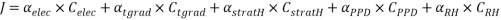The coefficients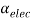,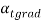,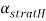,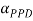, and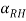in the above function are the weight factors for their related penalty costs. The weight factors values are set according to the system parameter. For the current system,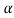‘s are set to unity.

1. electrical cost

The objective function that is to be optimized is the total operational cost of the system; this cost may be divided into:

• The cost of running the chiller.
• The cost of running the pump.
• The cost of running the fan.

Note that in this work the cost is given in units of KW.

#### Chiller Cost

The chiller is the main energy consuming component in our system .The chiller cost is expressed in terms of the part load ratio. The part load ratio is defined as the ratio of the current load on the chiller divided by the design load that the chiller could handle. Mathematically, the part load ratio is found from the equation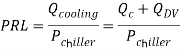The coefficient of performance of the chiller is correlated to the load equation by using the following correlation: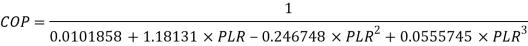The cost of the chiller is calculated by using the following equation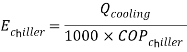1. Fan cost

The fan cost is directly related to the air mass flow rate by using the following equation: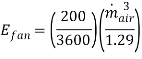1. Pump cost

The pump cost is related to the pump head, liquid desiccant mass flow rate, and the efficiency of the pump. The power of the pump is evaluated by multiplying the pressure difference by the volumetric flow rate and dividing the result by the pump efficiency; mathematically the pump cost equation may be written as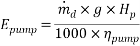Note that the pump cost is not included in the cost function, since the desiccant mass flow rate is costant.

Therefore the total energy consumed can be expressed by the following equation: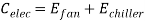### The Constraints Cost Functions

The cost function for the constraints may be written such that they could be incorporated into the online cost function in a simple manner. These constraints are related to their respective threshold values such that when the constraints are violated, the fitness function would have a very large value.

1. For the predicted person dissatisfied, the cost function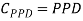1. The relative humidity cost function may be bounded from the upper side by using the relation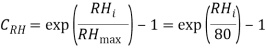1. The stratification height cost is bounded to be larger than 1m, thus the stratification height cost is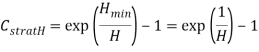1. The temperature gradient is to bounded to be less than 2.5 K/m, thus the temperature gradient cost function may be written as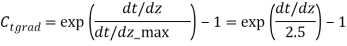The exponential term helps to penalize the cost function when-ever the thermal comfort level of occupants in the room TH decreases below the minimum set value THmin. This will increase the value of the cost function dramatically and the set of variables at hand is rejected. The integration of the constraint terms within the objective function expression and the use of the exponential form to control the constraints’ cost were implemented by Keblawi et al.  and Hammoud et al. .

View all

## DMCA / Removal Request

If you are the original writer of this essay and no longer wish to have your work published on UKEssays.com then please:

Related Services

Related Lectures